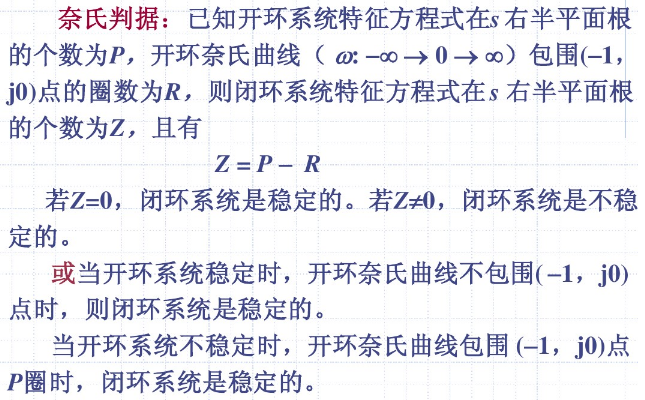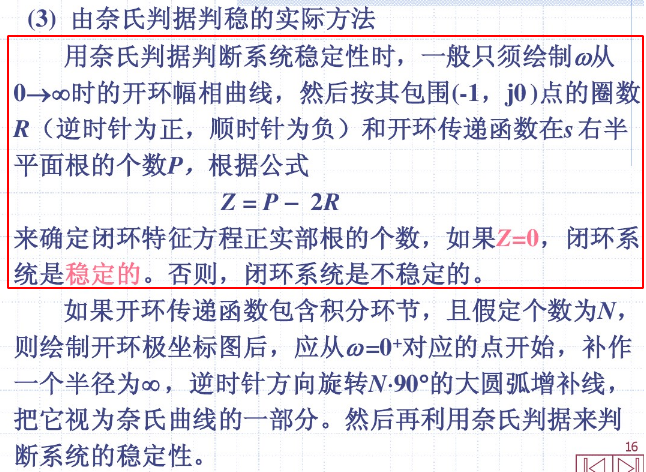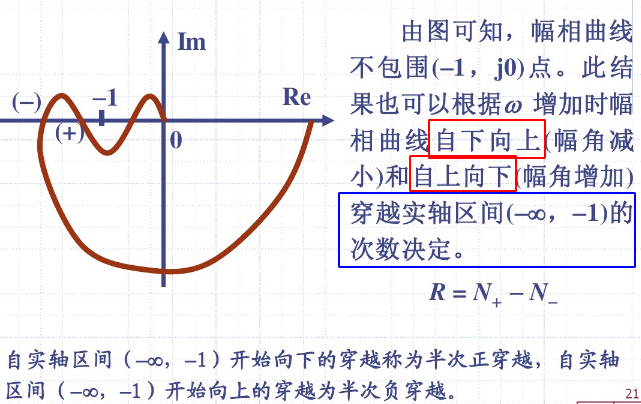# 1. 控制系统的李雅普诺夫稳定性分析

## 1.1. 对自动控制系统的要求

• 稳定：系统工作的前提
• 准确：稳态误差最小或者无差调节
• 快速：快速、平稳响应

## 1.2. 两种经典稳定性判据

### 1.2.2. 劳斯判据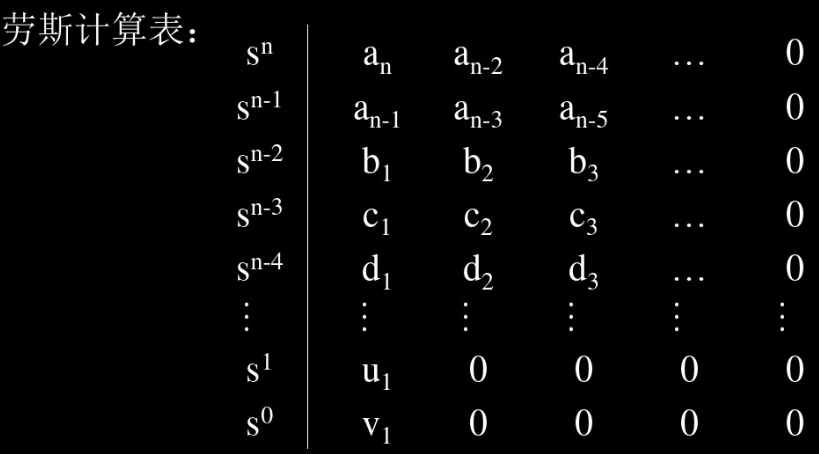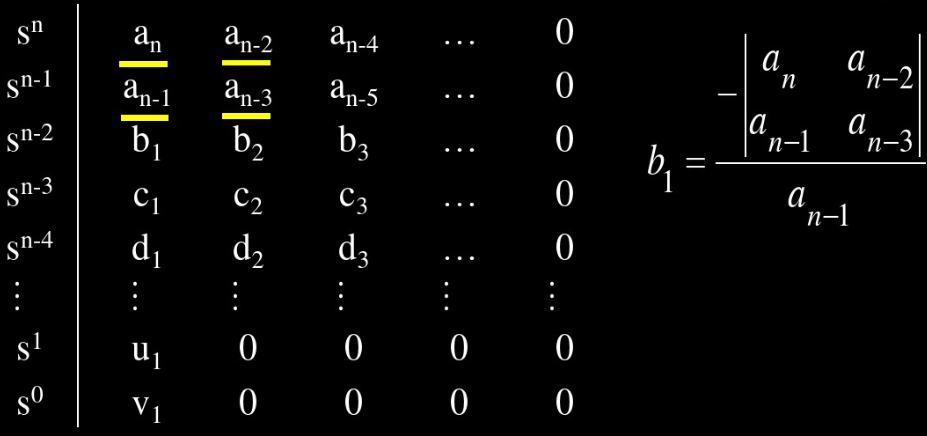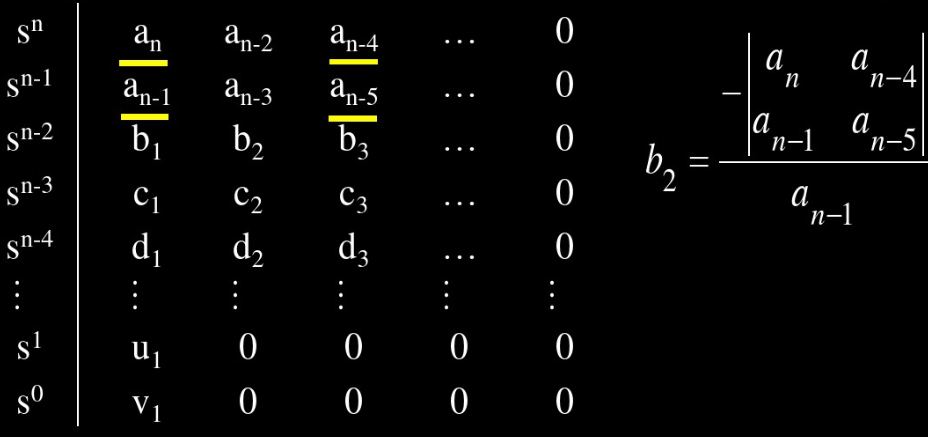# 2. 李雅普诺夫(Lyapunov)稳定性分析方法

## 2.1. 间接法——第一法

• 将非线性状态方程线性化
• 解线性化定长系统的特征值========>判定是否稳定

### 2.1.1. 推导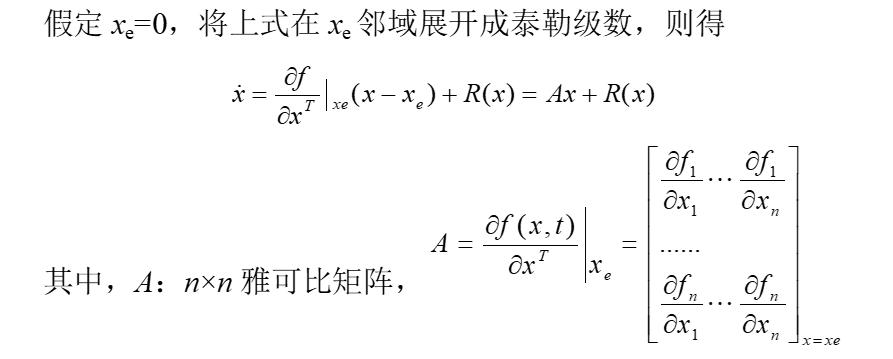• R(x)是包含对x的二次以及二次以上的高阶导数项，Ax为线性化之后的一次近似

• 如果矩阵A的所有特征值都具有负实部，则$$x_e$$总是渐近稳定的，而且系统的稳定性与高阶导数项R(x)无关
• 如果矩阵A的特征值中，至少有一个具有正的实部，则无论高阶项R(x)如何，$$x_e$$总是不稳定的
• 如果矩阵A的特征值中，至少有一个的实部为0，则$$x_e$$具有局部稳定特性，此时原方程不能使用其一次近似$$Ax$$来表征，此时属于临界状况，此时局部稳定性取决于高阶导数项R(x)

### 2.1.2. 例子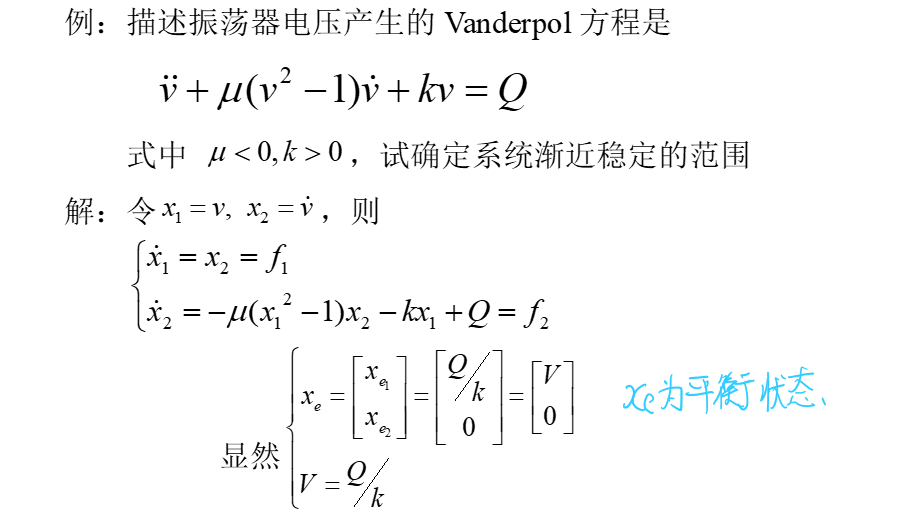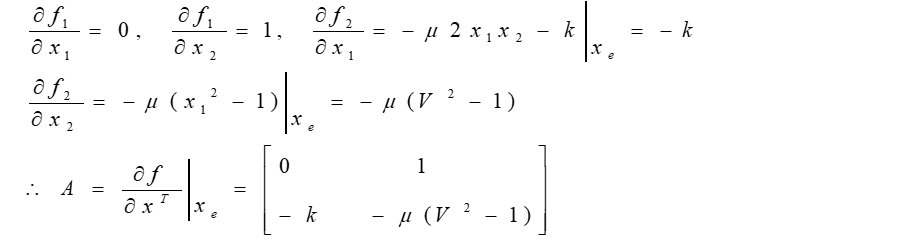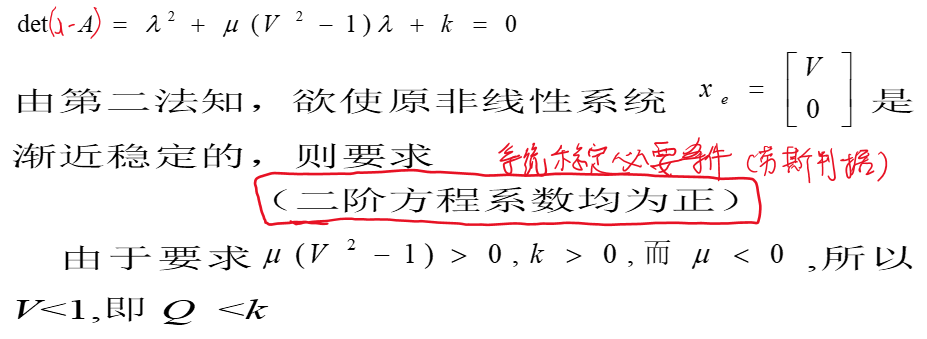## 2.2. 直接法——第二法

### 2.2.1. 先导知识——二次型及其定号性

#### 2.2.1.1. 二次型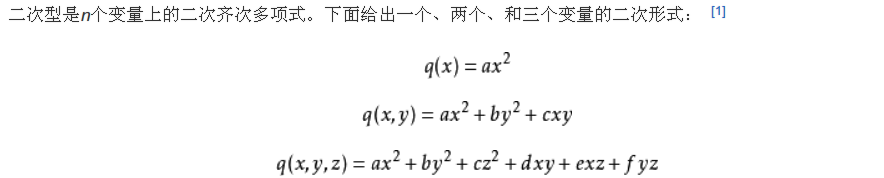#### 2.2.1.2. 正定二次型

$f(x_1,x_2,\cdots,x_n)=X^T A X$

\begin{aligned} \forall x \in R^n, X \neq 0 \end{aligned}

$f(x_1,x_2,\cdots,x_n)>0$

#### 2.2.1.3. 二次型标量函数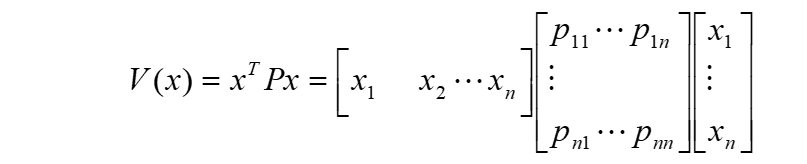#### 2.2.1.4. 定号性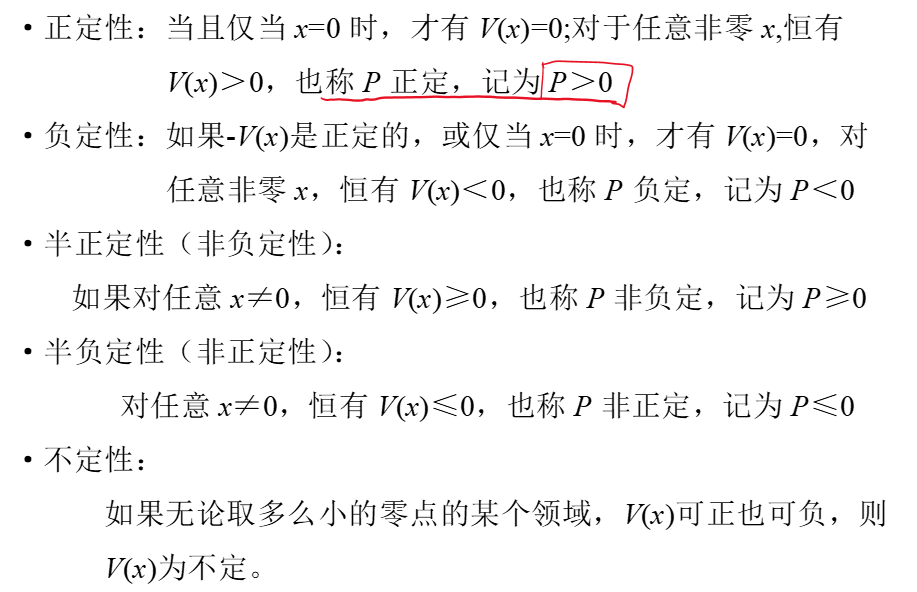#### 2.2.1.5. Sylvester(塞尔维斯特)准则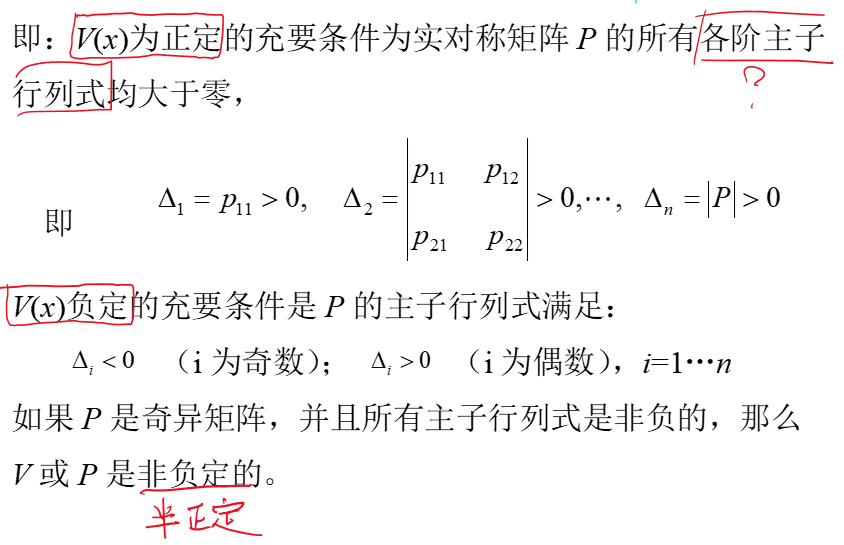#### 2.2.1.6. 矩阵A正定

• 正惯性指数(即正值的特征值个数)等于n
• 矩阵A与单位矩阵合同
• 矩阵A的顺序主子式大于0 (见后面补充)
• 矩阵A的特征值大于0

• 矩阵A的行列式大于0 (但是行列式大于0的矩阵不一定正定)

(1)顺序主子式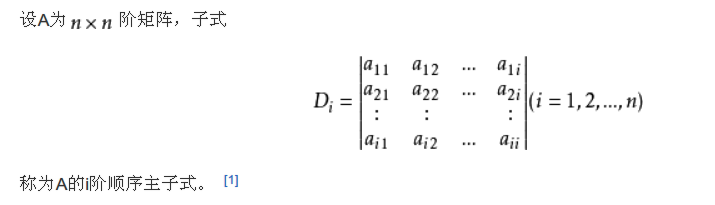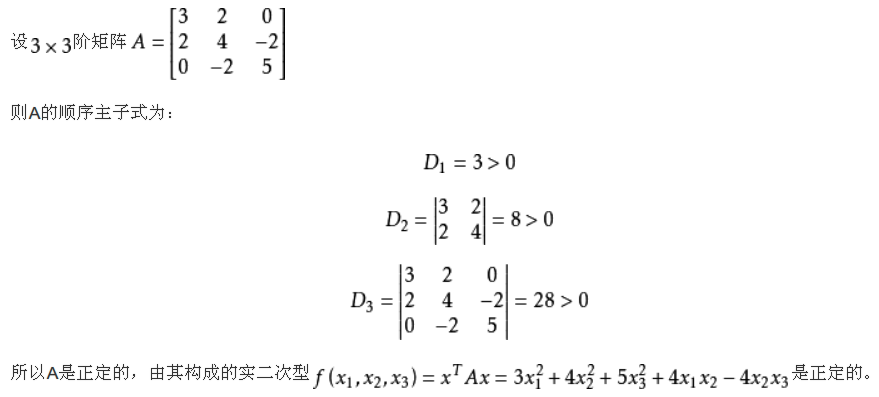### 2.2.2. 一致渐近稳定判定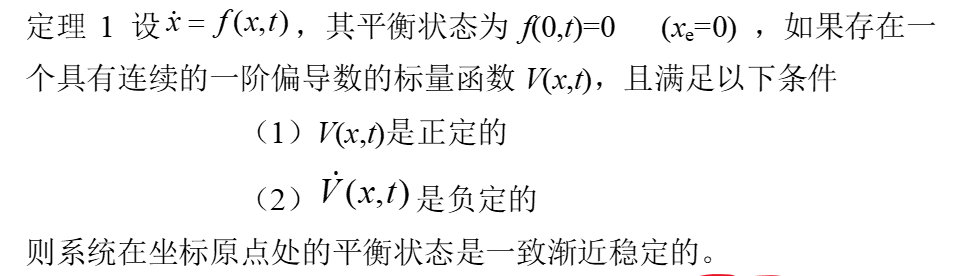#### 2.2.2.2. 李氏函数V(x,t)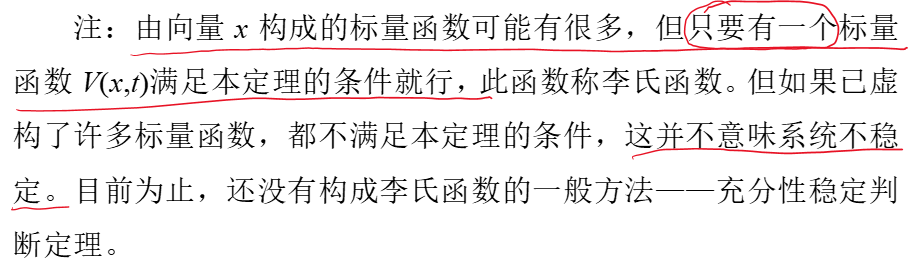#### 2.2.2.3. 形象描述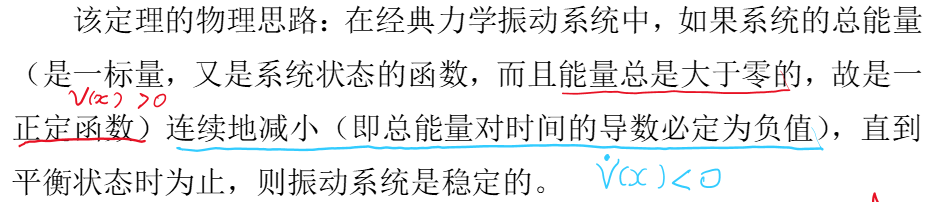### 2.2.3. 渐进稳定判定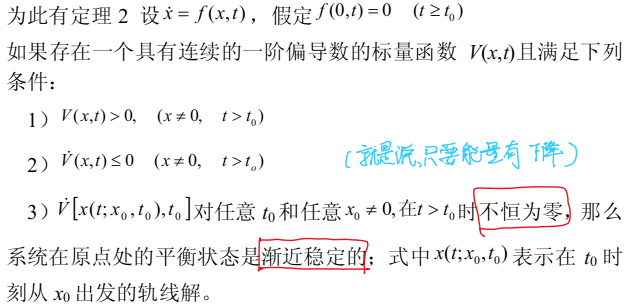### 2.2.4. 一致稳定(等幅震荡)(非渐近稳定)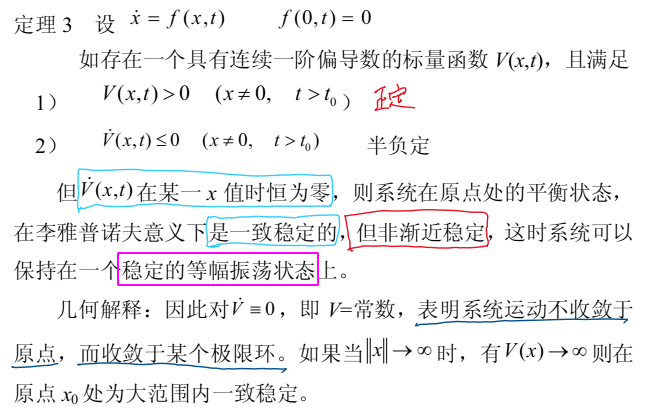### 2.2.5. 不稳定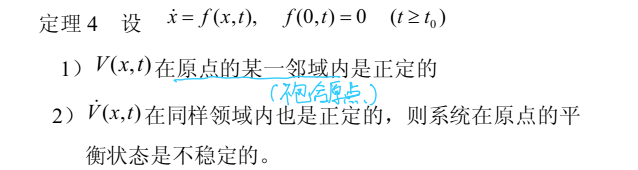### 2.2.6. 例子1(大范围渐近稳定)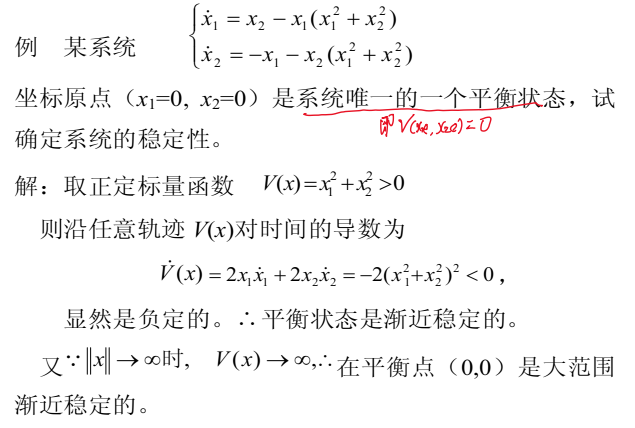### 2.2.7. 例子2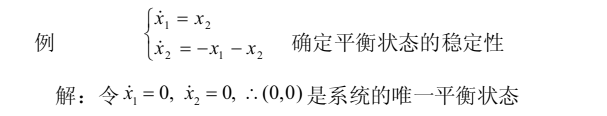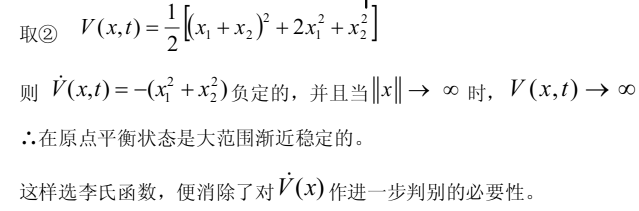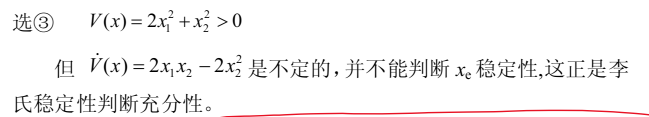### 2.2.8. 例子3(一致稳定)(等幅震荡)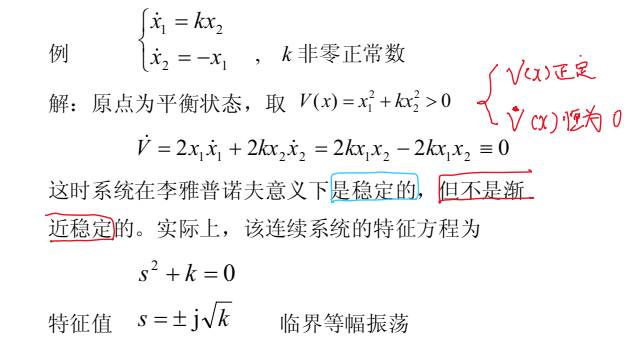### 2.2.9. 例子4(不稳定)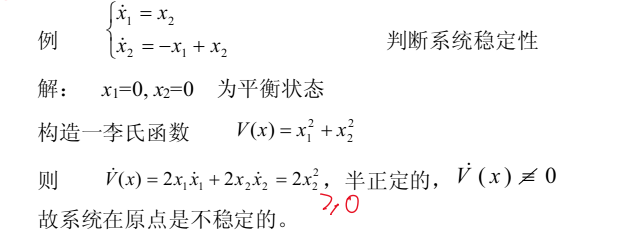# 3. 线性系统的李雅普诺夫稳定性分析

## 3.1. 线性定常系统(连续)

• 假设矩阵A为非奇异矩阵，则系统唯一平衡状态在原点$$x_e=0$$
• 如果它在状态空间中的某域内(包括$$x_e=0$$)是渐近稳定的，则它一定是大范围渐近稳定的
• 对线性系统$$\dot{x}=Ax$$，其李氏函数$$V(x)$$一定可以取为二次型$$x^TPx$$的形式。

### 3.1.1. 线性定常系统李氏函数的求法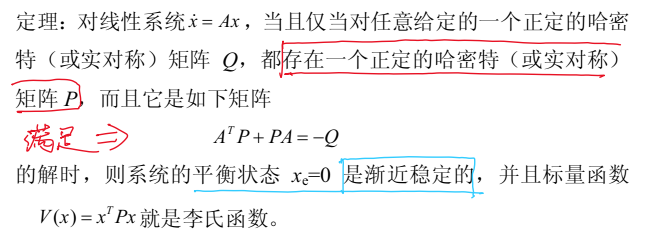### 3.1.2. 根据求法得到的推论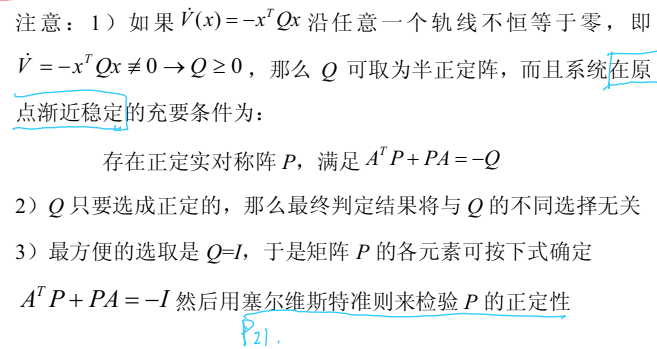### 3.1.3. 例子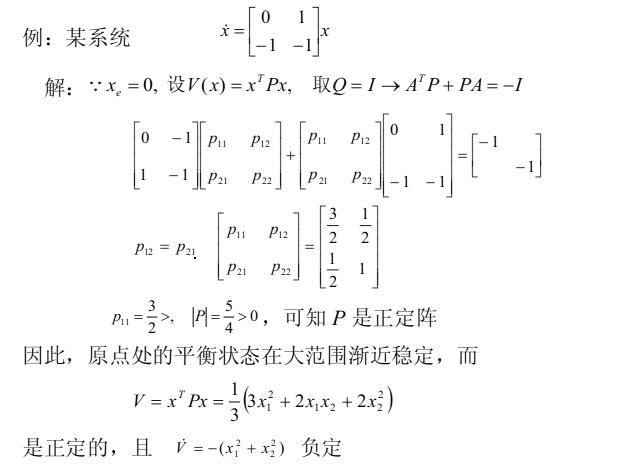## 3.2. 线性时变系统(连续)

### 3.2.1. 线性时变系统李氏函数的求法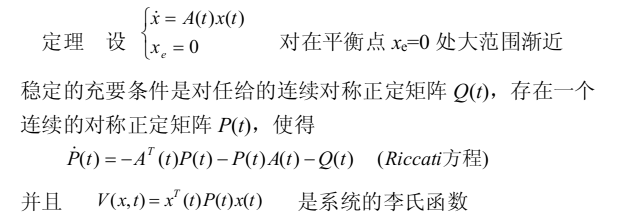## 3.3. 线性定常系统(离散)

$x(k+1)=Gx(k),~~~ x_e=0$

### 3.3.1. 线性定常(离散)系统李氏函数的求法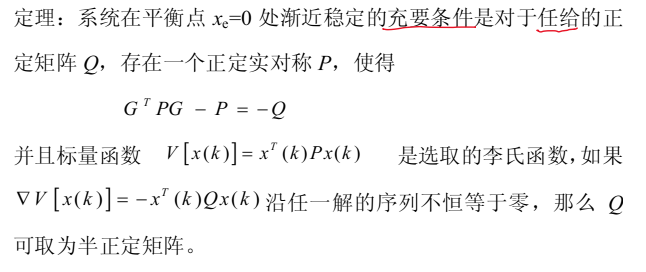### 3.3.2. 例子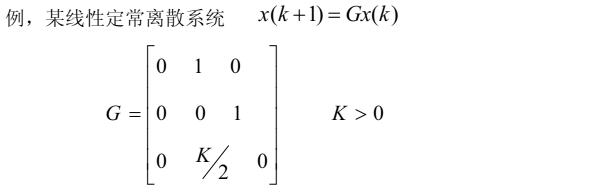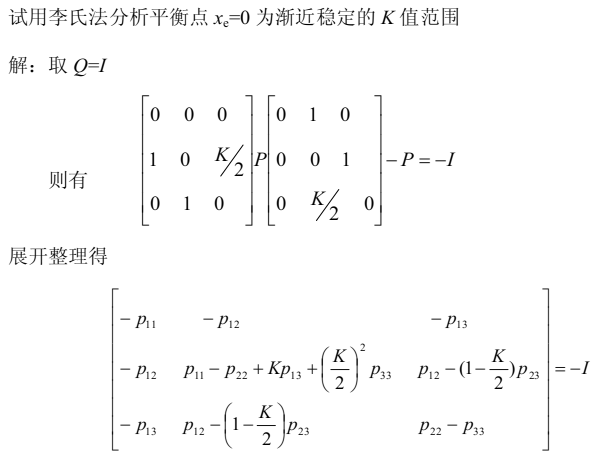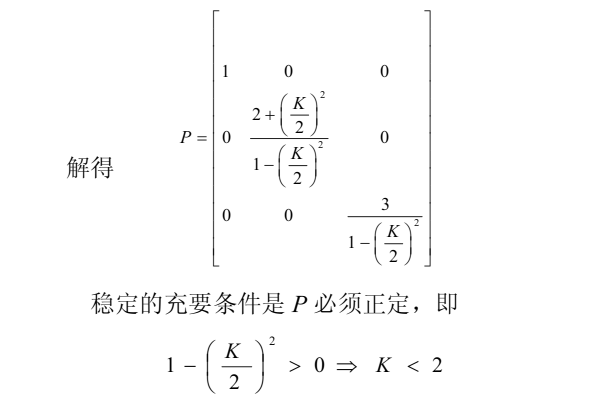## 3.4. 线性时变系统(离散)

$x(k+1)=G(k+1,k)x(k) ,~~~~ x_e=0$

### 3.4.1. 线性时变(离散)系统李氏函数的求法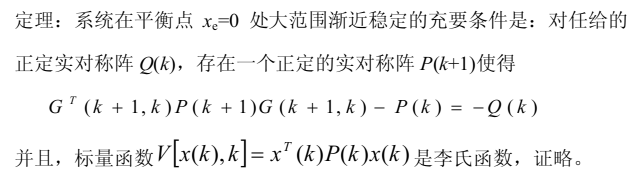# 4. 问答

## 4.1. 稳定性的一般定义

### 4.1.1. BIBO稳定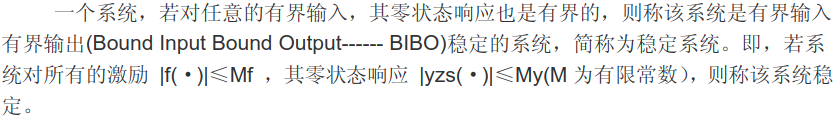### 4.1.2. 直观感受## 4.2. 线性定常系统稳定的充要条件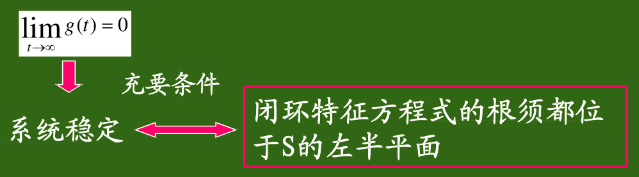### 4.2.1. 稳定性判据

#### 4.2.1.1. 直接解法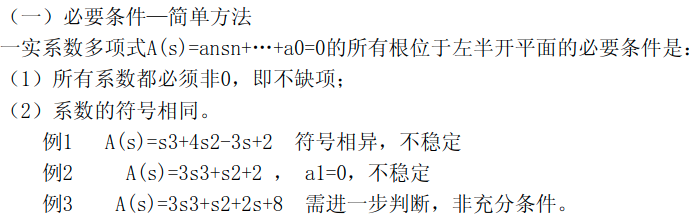#### 4.2.1.2. 赫尔维茨稳定判据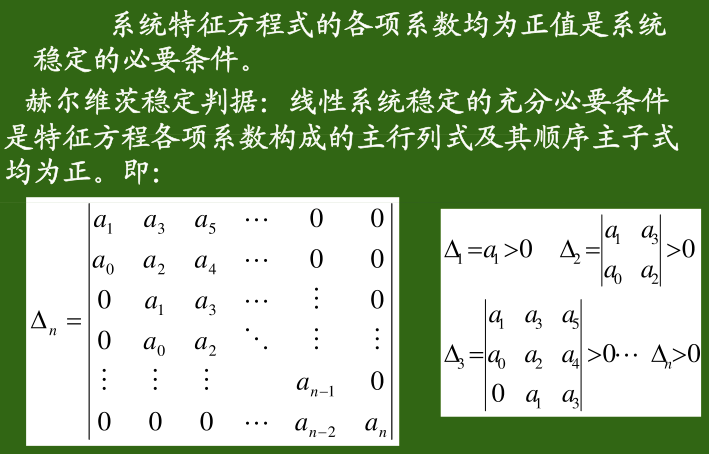#### 4.2.1.3. 劳斯判据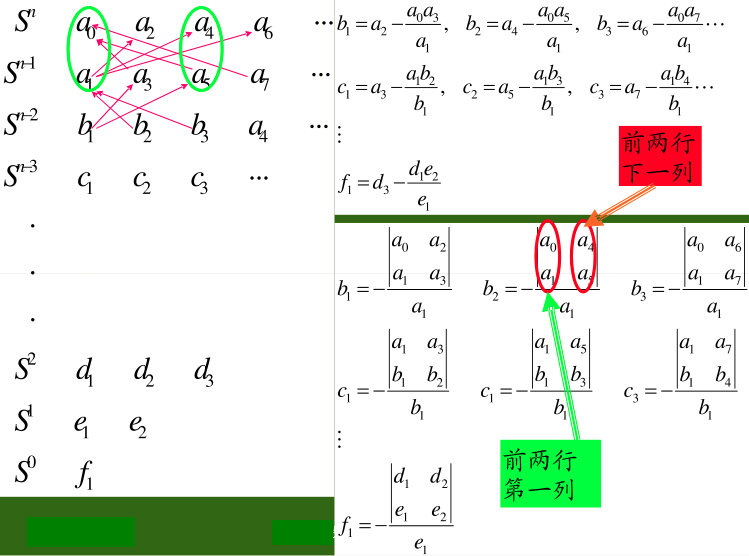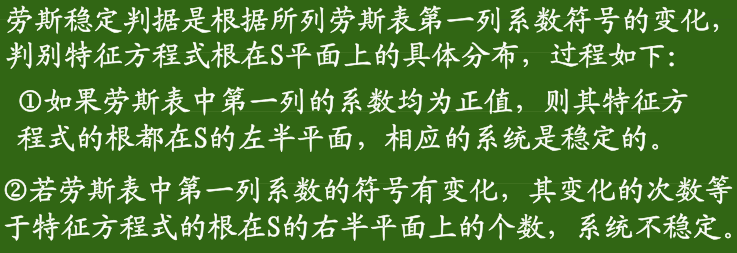1. 某一行第一项为0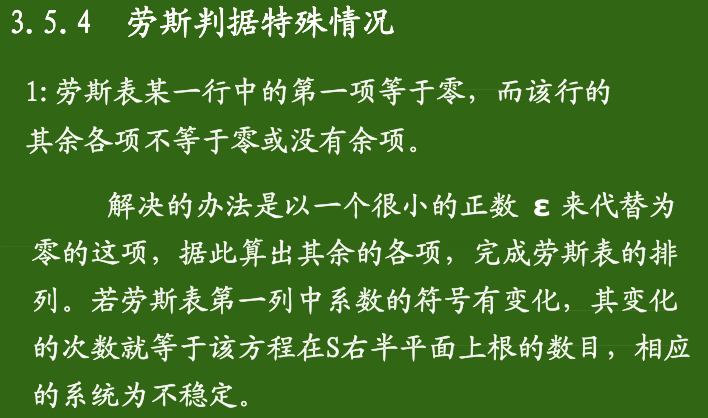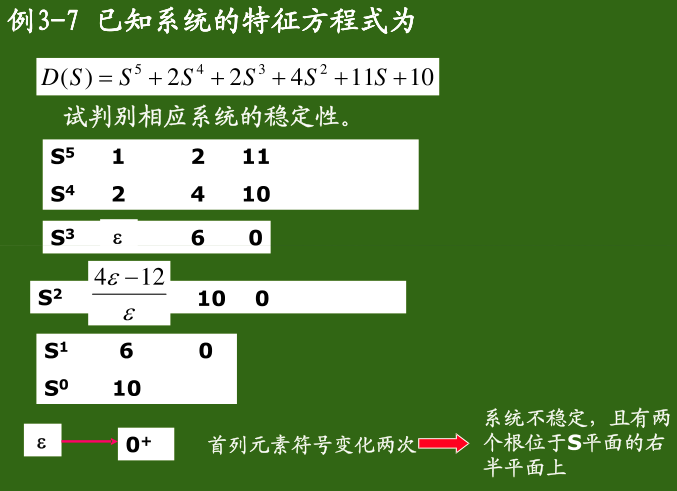1. 劳斯表出现全零行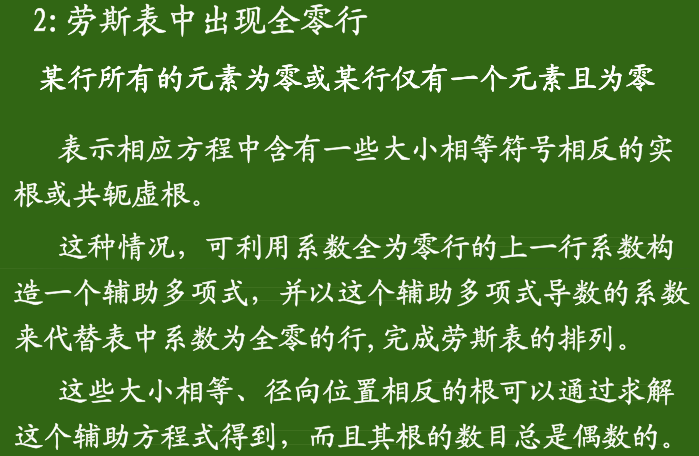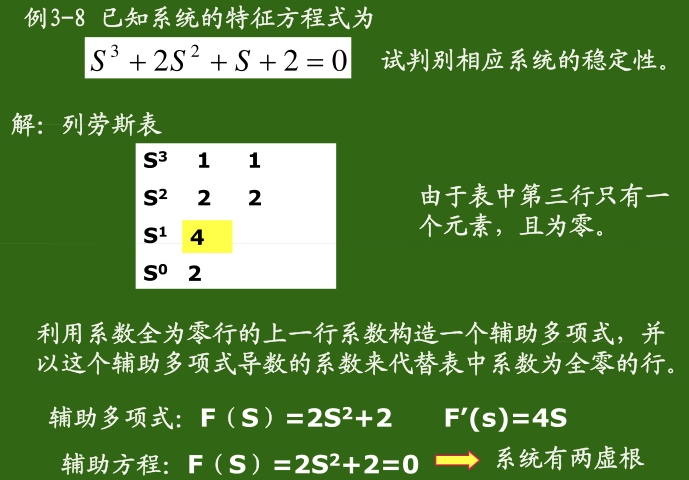#### 4.2.1.4. 奈奎斯特(Nyquist)准则